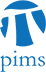## PIMS/AMI Seminar: The classical Sobolev inequalities: refinement and generalization

• Date: 02/09/2010
Lecturer(s):
Zhichun Zhai (UA)
Location:

University of Alberta

Description:

The classical Sobolev inequalities are important tools for partial differential equations, probability theory and geometric analysis. In this talk, I give an introduction to the area of the Sobolev inequalities. I will then discuss some new refinements and generalizations of the classical Sobolev inequalities. These inequalities include fractional-order Sobolev, Moser-Trudinger, Morrey-Sobolev inequalities under Lorentz norms, sharp affine Gagliardo-Nirenberg inequalities and $L^{p}-$Sobolev logarithmic inequalities. Here it is worth particularly mentioning that the fractional-order Sobolev type inequalities under Lebesgue norms are very useful in probability theory.

Schedule:

3:30pm, CAB 657.